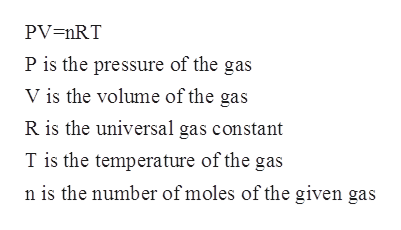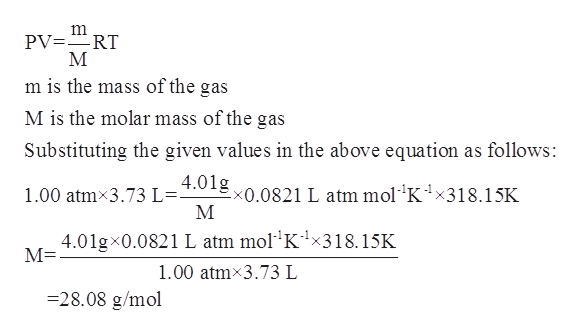# A 4.01 g sample of a certain diatomic gas occupies a volume of 3.73 L at 1.00 ATM and a temperature of 45°C. identify this gas

Question
100 views

A 4.01 g sample of a certain diatomic gas occupies a volume of 3.73 L at 1.00 ATM and a temperature of 45°C. identify this gas

check_circle

Step 1

The number of moles for a given chemical species refers to the ratio of mass of the species to that of its molar mass. The formula is shown below:

Step 2

The ideal gas equation is known as the general gas equation and is a equation of state for a given hypothetical gas. The equation is given by:help_outlineImage TranscriptionclosePV=nRT P is the pressure of the gas V is the volume of the gas R is the universal gas constant T is the temperature of the gas n is the number of moles of the given gas fullscreen
Step 3

In the given problem, the temperature is given to be 45oC =318.15 K.

The pressure of the gas is given to be 1.00 atm.

Volume occupied by the gas is given to be 3.73 L.

The...help_outlineImage Transcriptionclosem PV -RT М m is the mass of the gas M is the molar mass of the gas Substituting the given values in the above equation as follows: 4.01g 1.00 atm3.73 L=- x0.0821 L atm mol'K1x318.15K М 4.01gx0.0821 L atm mol"K-1x318.15K М- 1.00 atm 3.73 L 28.08 g/mol fullscreen

### Want to see the full answer?

See Solution

#### Want to see this answer and more?

Solutions are written by subject experts who are available 24/7. Questions are typically answered within 1 hour.*

See Solution
*Response times may vary by subject and question.
Tagged in

### Physical Chemistry